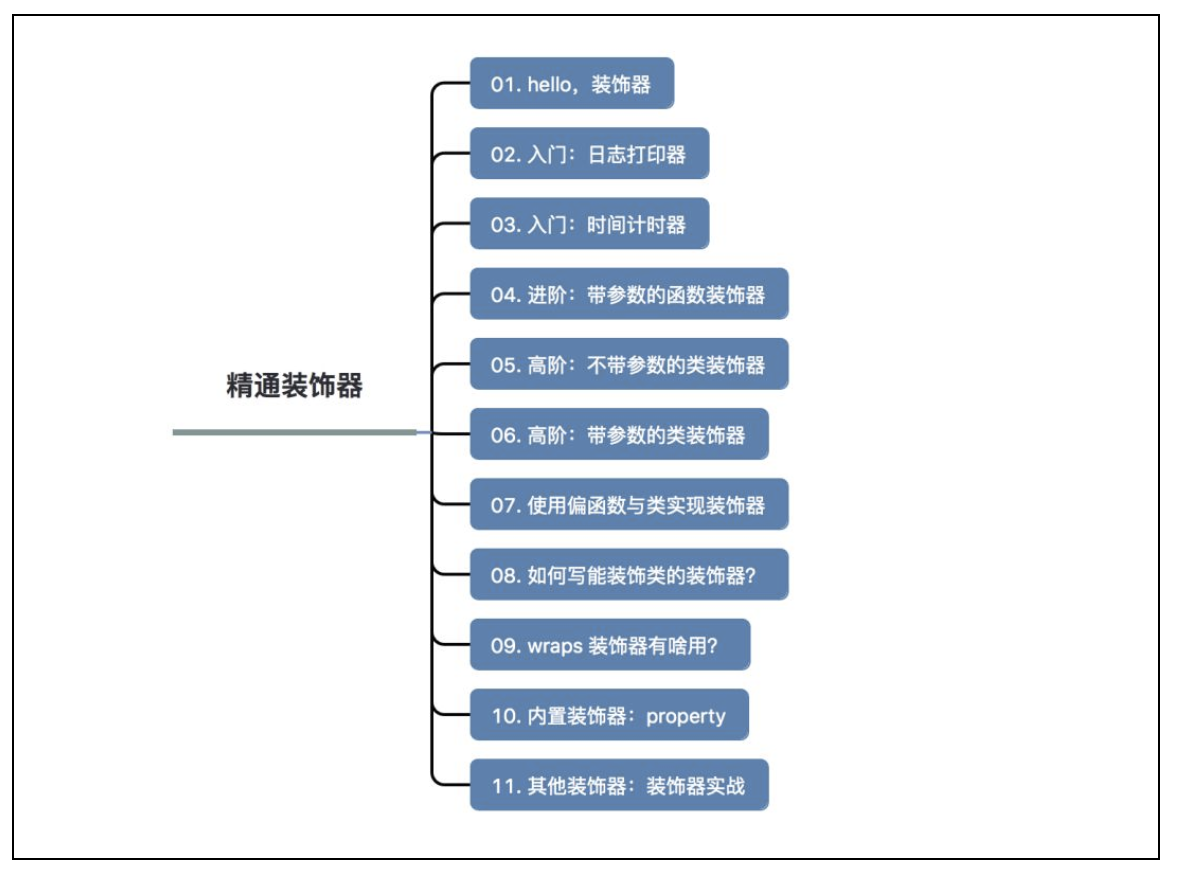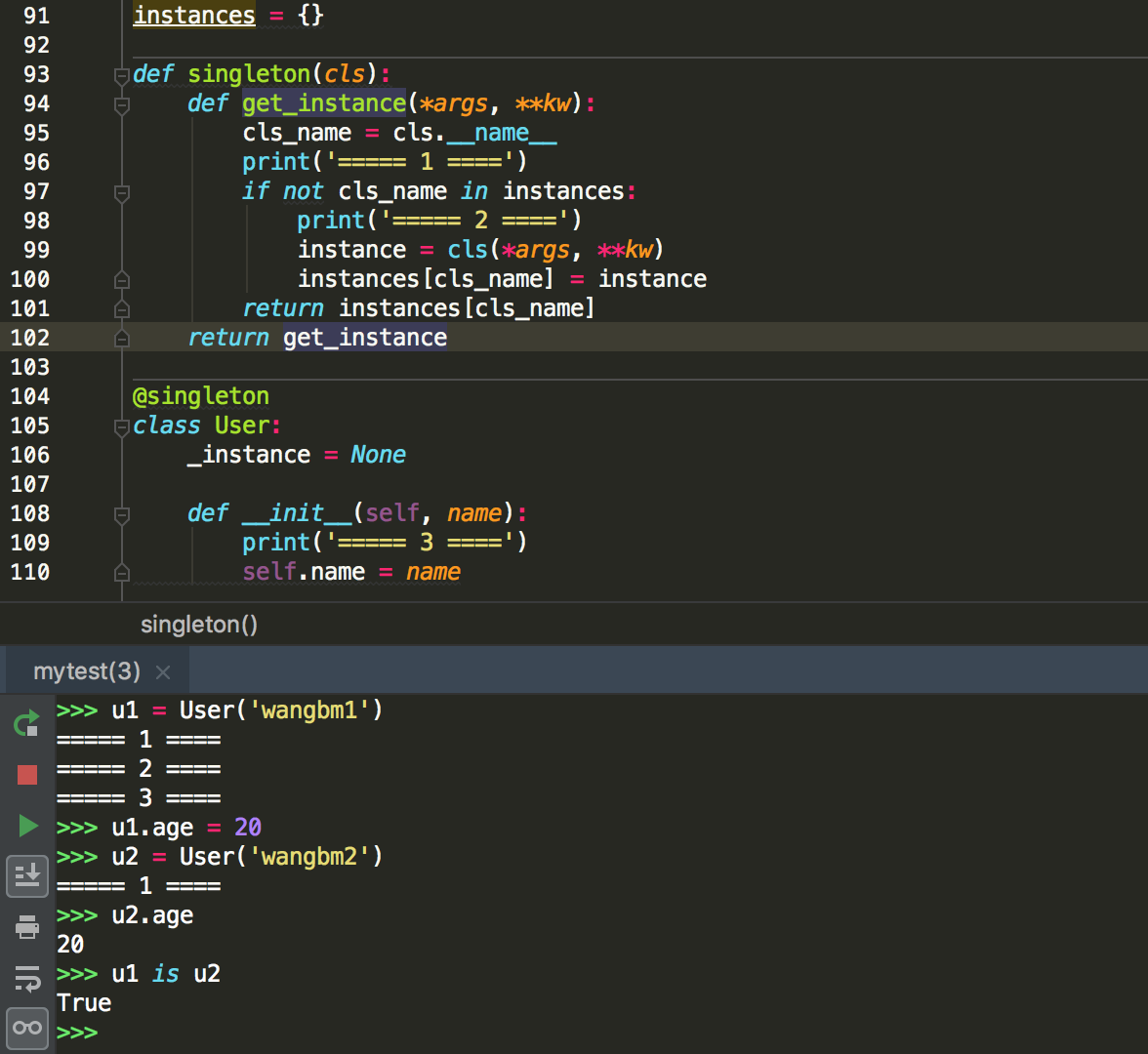# 3.1 装饰器进阶用法详解¶1、你都用过装饰器实现过什么样的功能？

2、如何写一个可以传参的装饰器？## 1. Hello，装饰器¶

def decorator(func):
def wrapper(*args, **kw):
return func()
return wrapper

@decorator
def function():
print("hello, decorator")


• 更加优雅，代码结构更加清晰

• 将实现特定的功能代码封装成装饰器，提高代码复用率，增强代码可读性

## 2. 入门：日志打印器¶

# 这是装饰器函数，参数 func 是被装饰的函数
def logger(func):
def wrapper(*args, **kw):
print('主人，我准备开始执行：{} 函数了:'.format(func.__name__))

# 真正执行的是这行。
func(*args, **kw)

print('主人，我执行完啦。')
return wrapper


@logger
print('{} + {} = {}'.format(x, y, x+y))


add(200, 50)


主人，我准备开始执行：add 函数了:
200 + 50 = 250



## 3. 入门：时间计时器¶

# 这是装饰函数
def timer(func):
def wrapper(*args, **kw):
t1=time.time()
# 这是函数真正执行的地方
func(*args, **kw)
t2=time.time()

# 计算下时长
cost_time = t2-t1
print("花费时间：{}秒".format(cost_time))
return wrapper


import time

@timer
def want_sleep(sleep_time):
time.sleep(sleep_time)

want_sleep(10)


花费时间：10.0073800086975098秒


## 4. 进阶：带参数的函数装饰器¶

@periodic_task(spacing=60)
def send_mail():
pass

def ntp()
pass


# 小明，中国人
@say_hello("china")
def xiaoming():
pass

# jack，美国人
@say_hello("america")
def jack():
pass


def say_hello(contry):
def wrapper(func):
def deco(*args, **kwargs):
if contry == "china":
print("你好!")
elif contry == "america":
print('hello.')
else:
return

# 真正执行函数的地方
func(*args, **kwargs)
return deco
return wrapper


xiaoming()
print("------------")
jack()


你好!
------------
hello.


## 5. 高阶：不带参数的类装饰器¶

class logger(object):
def __init__(self, func):
self.func = func

def __call__(self, *args, **kwargs):
print("[INFO]: the function {func}() is running..."\
.format(func=self.func.__name__))
return self.func(*args, **kwargs)

@logger
def say(something):
print("say {}!".format(something))

say("hello")


[INFO]: the function say() is running...
say hello!


## 6. 高阶：带参数的类装饰器¶

__init__ ：不再接收被装饰函数，而是接收传入参数。 __call__ ：接收被装饰函数，实现装饰逻辑。

class logger(object):
def __init__(self, level='INFO'):
self.level = level

def __call__(self, func): # 接受函数
def wrapper(*args, **kwargs):
print("[{level}]: the function {func}() is running..."\
.format(level=self.level, func=func.__name__))
func(*args, **kwargs)
return wrapper  #返回函数

@logger(level='WARNING')
def say(something):
print("say {}!".format(something))

say("hello")


[WARNING]: the function say() is running...
say hello!


## 7. 使用偏函数与类实现装饰器¶

import time
import functools

class DelayFunc:
def __init__(self,  duration, func):
self.duration = duration
self.func = func

def __call__(self, *args, **kwargs):
print(f'Wait for {self.duration} seconds...')
time.sleep(self.duration)
return self.func(*args, **kwargs)

def eager_call(self, *args, **kwargs):
print('Call without delay')
return self.func(*args, **kwargs)

def delay(duration):
"""
装饰器：推迟某个函数的执行。
同时提供 .eager_call 方法立即执行
"""
# 此处为了避免定义额外函数，
# 直接使用 functools.partial 帮助构造 DelayFunc 实例
return functools.partial(DelayFunc, duration)


@delay(duration=2)
return a+b


>>> add    # 可见 add 变成了 Delay 的实例
<__main__.DelayFunc object at 0x107bd0be0>
>>>
Wait for 2 seconds...
8
>>>


## 8. 如何写能装饰类的装饰器？¶

instances = {}

def singleton(cls):
def get_instance(*args, **kw):
cls_name = cls.__name__
print('===== 1 ====')
if not cls_name in instances:
print('===== 2 ====')
instance = cls(*args, **kw)
instances[cls_name] = instance
return instances[cls_name]
return get_instance

@singleton
class User:
_instance = None

def __init__(self, name):
print('===== 3 ====')
self.name = name## 9. wraps 装饰器有啥用？¶

def wrapper(func):
def inner_function():
pass
return inner_function

@wrapper
def wrapped():
pass

print(wrapped.__name__)
#inner_function


def wrapper(func):
def inner_function():
pass
return inner_function

def wrapped():
pass

print(wrapper(wrapped).__name__)
#inner_function


from functools import wraps

def wrapper(func):
@wraps(func)
def inner_function():
pass
return inner_function

@wrapper
def wrapped():
pass

print(wrapped.__name__)
# wrapped


def wraps(wrapped,
assigned = WRAPPER_ASSIGNMENTS,
return partial(update_wrapper, wrapped=wrapped,
assigned=assigned, updated=updated)


from functools import update_wrapper

WRAPPER_ASSIGNMENTS = ('__module__', '__name__', '__qualname__', '__doc__',
'__annotations__')

def wrapper(func):
def inner_function():
pass

update_wrapper(inner_function, func, assigned=WRAPPER_ASSIGNMENTS)
return inner_function

@wrapper
def wrapped():
pass

print(wrapped.__name__)


## 10. 内置装饰器：property¶

class Student(object):
def __init__(self, name, age=None):
self.name = name
self.age = age

# 实例化
xiaoming = Student("小明")

# 添加属性
xiaoming.age=25

# 查询属性
xiaoming.age

# 删除属性
del xiaoming.age


class Student(object):
def __init__(self, name):
self.name = name
self.name = None

def set_age(self, age):
if not isinstance(age, int):
raise ValueError('输入不合法：年龄必须为数值!')
if not 0 < age < 100:
raise ValueError('输入不合法：年龄范围必须0-100')
self._age=age

def get_age(self):
return self._age

def del_age(self):
self._age = None

xiaoming = Student("小明")

# 添加属性
xiaoming.set_age(25)

# 查询属性
xiaoming.get_age()

# 删除属性
xiaoming.del_age()


# 赋值
xiaoming.age = 25

# 获取
xiaoming.age


class Student(object):
def __init__(self, name):
self.name = name
self.name = None

@property
def age(self):
return self._age

@age.setter
def age(self, value):
if not isinstance(value, int):
raise ValueError('输入不合法：年龄必须为数值!')
if not 0 < value < 100:
raise ValueError('输入不合法：年龄范围必须0-100')
self._age=value

@age.deleter
def age(self):
del self._age

xiaoming = Student("小明")

# 设置属性
xiaoming.age = 25

# 查询属性
xiaoming.age

# 删除属性
del xiaoming.age


@property装饰过的函数，会将一个函数定义成一个属性，属性的值就是该函数return的内容。同时，会将这个函数变成另外一个装饰器。就像后面我们使用的@age.setter@age.deleter

@age.setter 使得我们可以使用XiaoMing.age = 25这样的方式直接赋值。 @age.deleter 使得我们可以使用del XiaoMing.age这样的方式来删除属性。

property 的底层实现机制是「描述符」，为此我还写过一篇文章。

class Student:
def __init__(self, name):
self.name = name

@property
def math(self):
return self._math

@math.setter
def math(self, value):
if 0 <= value <= 100:
self._math = value
else:
raise ValueError("Valid value must be in [0, 100]")


class TestProperty(object):

def __init__(self, fget=None, fset=None, fdel=None, doc=None):
self.fget = fget
self.fset = fset
self.fdel = fdel
self.__doc__ = doc

def __get__(self, obj, objtype=None):
print("in __get__")
if obj is None:
return self
if self.fget is None:
raise AttributeError
return self.fget(obj)

def __set__(self, obj, value):
print("in __set__")
if self.fset is None:
raise AttributeError
self.fset(obj, value)

def __delete__(self, obj):
print("in __delete__")
if self.fdel is None:
raise AttributeError
self.fdel(obj)

def getter(self, fget):
print("in getter")
return type(self)(fget, self.fset, self.fdel, self.__doc__)

def setter(self, fset):
print("in setter")
return type(self)(self.fget, fset, self.fdel, self.__doc__)

def deleter(self, fdel):
print("in deleter")
return type(self)(self.fget, self.fset, fdel, self.__doc__)


class Student:
def __init__(self, name):
self.name = name

# 其实只有这里改变
@TestProperty
def math(self):
return self._math

@math.setter
def math(self, value):
if 0 <= value <= 100:
self._math = value
else:
raise ValueError("Valid value must be in [0, 100]")


1. 使用TestProperty装饰后，math 不再是一个函数，而是TestProperty 类的一个实例。所以第二个math函数可以使用 math.setter 来装饰，本质是调用TestProperty.setter 来产生一个新的 TestProperty 实例赋值给第二个math

2. 第一个 math 和第二个 math 是两个不同 TestProperty 实例。但他们都属于同一个描述符类（TestProperty），当对 math 对于赋值时，就会进入 TestProperty.__set__，当对math 进行取值里，就会进入 TestProperty.__get__。仔细一看，其实最终访问的还是Student实例的 _math 属性。

# 运行后，会直接打印这一行，这是在实例化 TestProperty 并赋值给第二个math
in setter
>>>
>>> s1.math = 90
in __set__
>>> s1.math
in __get__
90


## 11. 其他装饰器：装饰器实战¶

import signal

class TimeoutException(Exception):
def __init__(self, error='Timeout waiting for response from Cloud'):
Exception.__init__(self, error)

def timeout_limit(timeout_time):
def wraps(func):
def handler(signum, frame):
raise TimeoutException()

def deco(*args, **kwargs):
signal.signal(signal.SIGALRM, handler)
signal.alarm(timeout_time)
func(*args, **kwargs)
signal.alarm(0)
return deco
return wraps